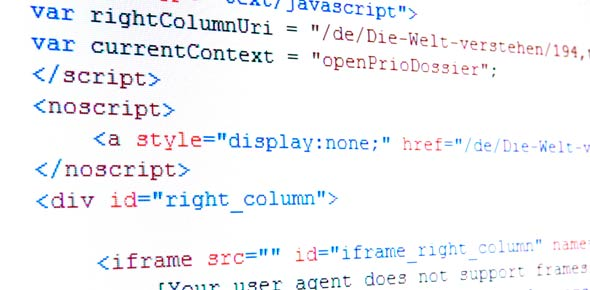# It's All About Python Programming Language Quiz

20 Questions | Total Attempts: 166SettingsThese questions are based on introduction about python programming language. Let's take this quiz and learn about it!

Related Topics
• 1.
What will the following Python statements output?print("Answer = ", 2*5)
• 2.
What will the following Python statements output?print("Age:", "20+5")
• 3.
What will the following Python statements output?print(float(34+5))
• 4.
What will the following Python statements output?print(int(6.9))
• 5.
• print("Answer = ", 2+5) _____________________
• 6.
•  print("Age:", "20") _________________________
• 7.
Print(int(30.5+4.2)) _________________________
• 8.
Print(float(6)) _________________________
• 9.
What do you think the following Python statements outputprint("Age:", 20) _________________________
• 10.
• What do you think the following Python statements outputprint(float(35-18)) _________________________
• 11.
What do you think the following Python statements outputprint(int(8/3)) _________________________
• 12.
• 13.
In the following program segment, what is the final output and how many times will the program loop be performed?count = 1while count < 9:print(count)count = count +2
• A.

Last Output: 9, loop: 9

• B.

Last Output: 8, loop: 8

• C.

Last Output: 9, loop: 8

• D.

The program will not execute

• 14.
What will be the output of the following program segment?aNum = 123aString = 2*str(aNum)print (aString)
• A.

246

• B.

246246

• C.

123123

• D.

'123123'

• 15.
• Ade has written the following pseudocode to multiply all positive integers less than 3. Is there any error in the pseudocode?x ← 0i ← 1While (i<3)x ← x * ii ← i+1
• A.

The initial value of x should be 1

• B.

i

• C.

There should be read statement for x

• D.

No error

• 16.
• What will be the final value of the variable count in the following pseudocode?count ← 1sum ← 0while(count<5)input numberSum = sum + numbercount = count +1
• A.

4

• B.

5

• C.

79

• D.

9

• 17.
Which of the following code is correct?
• A.

Print("Programming is fun") print("Python is fun")

• B.

Print('Programming is fun') print('Python is fun')

• C.

None

• D.

Both

• 18.
Print (7-6/2*3+ 10) will give output_____________ on Java
• A.

8.0

• B.

8.90

• C.

8

• D.

11.5

• 19.
If A = 1.0, B = 1.0 and C = 2.0, find the value of X inX = (4*B - B^2)/(2-A)
• A.

1.0

• B.

1.5

• C.

3.0

• D.

5.0

• 20.
If A = 1.0, B = 1.0 and C = 2.0, find the value of X inX = (2*A+B)+C/2*C
• A.

4.0

• B.

5.0

• C.

6.0

• D.

8.0